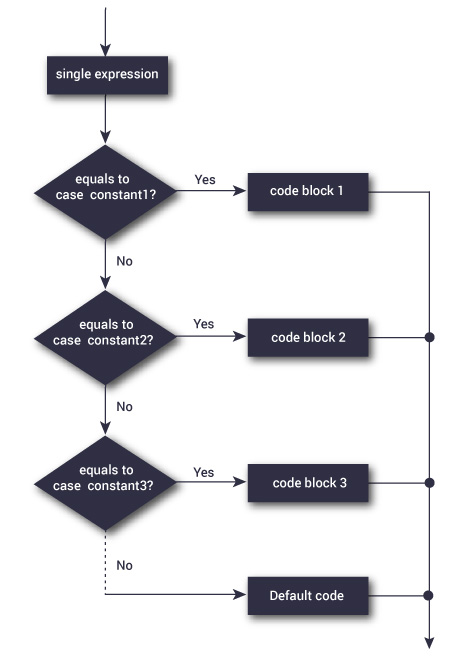# C++ Switch Case Statement

The nested `if...else` statement allows you to execute a block code among many alternatives. If you are checking on the value of a single variable in nested if…else statement, it is better to use switch statement.

The switch statement is often faster than nested if…else (not always). Also, the syntax of switch statement is cleaner and easier to understand.

## C++ switch…case syntax

```switch (n)
{
case constant1:
// code to be executed if n is equal to constant1;
break;

case constant2:
// code to be executed if n is equal to constant2;
break;
.
.
.
default:
// code to be executed if n doesn't match any constant
}
```

When a case constant is found that matches the switch expression, control of the program passes to the block of code associated with that case.

In the above pseudocode, suppose the value of n is equal to constant2. The compiler will execute the block of code associated with the case statement until the end of switch block, or until the break statement is encountered.

The break statement is used to prevent the code running into the next case.

## Flowchart of switch Statement## Example: C++ switch Statement

``````// Program to built a simple calculator using switch Statement

#include <iostream.h>
using namespace std;

int main()
{
char o;
float num1, num2;

cout << "Enter an operator (+, -, *, /): ";
cin >> o;

cout << "Enter two operands: ";
cin >> num1 >> num2;

switch (o)
{
case '+':
cout << num1 << " + " << num2 << " = " << num1+num2;
break;
case '-':
cout << num1 << " - " << num2 << " = " << num1-num2;
break;
case '*':
cout << num1 << " * " << num2 << " = " << num1*num2;
break;
case '/':
cout << num1 << " / " << num2 << " = " << num1/num2;
break;
default:
// operator is doesn't match any case constant (+, -, *, /)
cout << "Error! operator is not correct";
break;
}

return 0;
}``````

Output

```Enter an operator (+, -, *, /): +
-
Enter two operands: 2.3
4.5
2.3 - 4.5 = -2.2
```

The – operator entered by the user is stored in o variable. And, two operands 2.3 and 4.5 are stored in variables num1 and num2 respectively.

Then, the control of the program jumps to

`cout << num1 << " - " << num2 << " = " << num1-num2;`

Finally, the break statement ends the switch statement.

If break statement is not used, all cases after the correct case is executed.